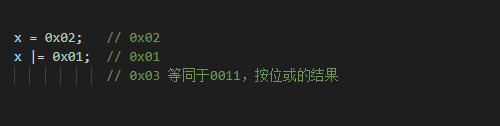1、C语言中的 >>= 意思为：右移后bai赋值

x = 8;
x >>= 3;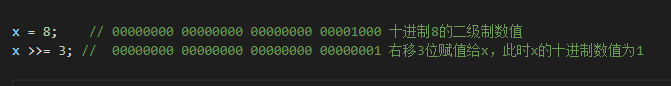2、C语言中的 <<= 意思为：左移后赋值

x = 8;

x <<= 3;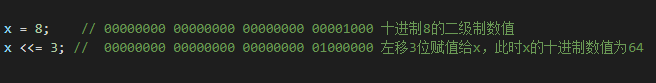3、C语言中的 &= 意思为：按位与后赋值

x = 0x02;

x &= 0x01;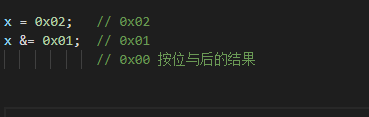4、C语言中的 ^= 意思为：按位异或后赋值

x = 0x02;

x ^= 0x01;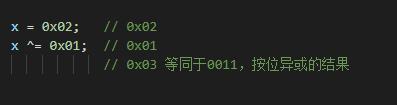5、C语言中的 |= 意思为：按位或后赋值

x = 0x02;

x  |= 0x01;